MATLAB Function Referenceacos

Inverse cosine, result in radians

Syntax

• ```Y = acos(X)
```

Description

```Y = acos(X) ``` returns the inverse cosine (arccosine) for each element of `X`. For real elements of `X` in the domain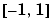, `acos(X)` is real and in the range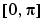. For real elements of `X` outside the domain, `acos(X)` is complex.

The `acos` function operates element-wise on arrays. The function's domains and ranges include complex values. All angles are in radians.

Examples

Graph the inverse cosine function over the domain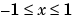.

• ```x = -1:.05:1;
plot(x,acos(x)), grid on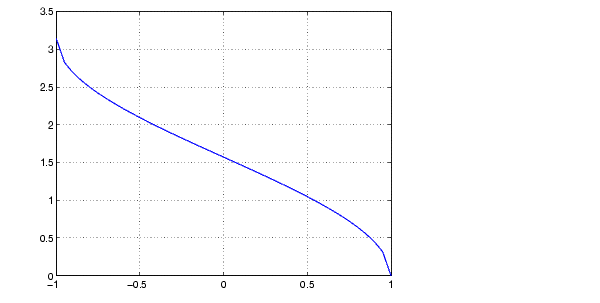```

Definition

The inverse cosine can be defined as

•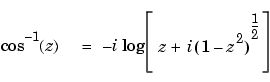Algorithm

`acos` uses FDLIBM, which was developed at SunSoft, a Sun Microsystems, Inc. business, by Kwok C. Ng, and others. For information about FDLIBM, see http://www.netlib.org.

See Also

`acosd`, `acosh`, `cos`

© 1994-2005 The MathWorks, Inc.